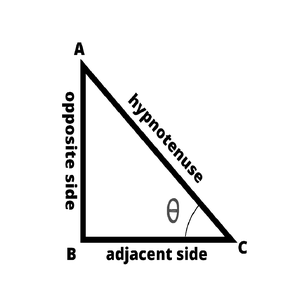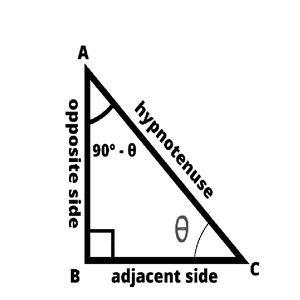# How do I find the value of sin 150° in fraction form?

• Difficulty Level : Basic
• Last Updated : 26 Nov, 2021

Trigonometry is a branch in mathematics that relates the sides of the triangle to the angles. Using some ratios, relations, and identities for a triangle in terms of its sides and its corresponding angles, many problems(distance, height, etc) can be solved and calculated easily and intuitively. There are several standard ratios or relations between the angles and the sides of the triangle that help to solve some basic as well as complex problems.

### Trigonometric Ratios

A Trigonometric ratio is defined as the proportion of the sides to the angles of a right-angled triangle. A standard trigonometric ratio can be obtained as the ratio of the sides to either of the acute angles of the right-angled triangles. Some definitions of some standard trigonometric ratios such as sine, cosine, and tangent are as follows,

Hey! Looking for some great resources suitable for young ones? You've come to the right place. Check out our self-paced courses designed for students of grades I-XII

Start with topics like Python, HTML, ML, and learn to make some games and apps all with the help of our expertly designed content! So students worry no more, because GeeksforGeeks School is now here!Sine is the function that takes in the parameter an angle θ, which is either of the acute-angles in the right-angled triangles and is defined as the ratio of the length of the opposite side to the hypotenuse of the right-angled triangle. In technical terms, it can be written as follows,

sin(θ) = opposite side / hypotenuse

Cosine is the function that takes in the parameter an angle θ, which is either of the acute-angles in the right-angled triangles and is defined as the ratio of the length of the adjacent side to the hypotenuse of the right-angled triangle. In technical terms, it can be written as follow,

cos(θ) = adjacent side / hypotenuse

Tangent is the function that takes in the parameter an angle θ, which is either of the acute-angles in the right-angled triangles and is defined as the ratio of the length of the opposite side to the adjacent side of the right-angled triangle. In technical terms, it can be written as follows,

tan(θ) = opposite side / adjacent side

These trigonometric ratios are related to one another using some trigonometric identities and formulas,

tan(θ) = sin(θ) / cos(θ)

sin²(θ) + cos²(θ) = 1

Each of the trigonometric ratios has other three derived trigonometric ratios which are deduced by taking the inverse of the respective ratios. The Other three Trigonometric ratios are Cosecant, Secant, and Cotangent used mathematically as cosec, sec, and cot. These are related to the Primary trigonometric ratios as follows,

cosec(θ) = 1 / sin(θ)

sec(θ) = 1 / cos(θ)

cot(θ) = 1 / tan(θ) = cos(θ) / sin(θ)

Below are some of the identities related to the standard trigonometric ratios and the derived trigonometric ratios,

tan²(θ) + 1 = sec²(θ)

cot²(θ) + 1 = cosec²(θ)

### Trigonometric Table

The below is the table for some common angles and the basic trigonometric ratios. The value of each angle in the trigonometry is fixed and known but the ones mentioned are more common and mostly used,

There are also some other trigonometric ratios to apply beyond the right-angled triangles:

sin(-θ) = – sin(θ)

cos(-θ) = cos(θ)

tan(-θ) = – tan(θ)

### Complementary and Supplementary angles

Certain formulas are defined for complementary and supplementary angles. The complementary angles are the angles that add up to form 90° or π/2 radians. We can form such angles and find the equivalent angles in terms of the trigonometric ratios.

The supplementary angles are the angles that add up to form 180° or π radians. We can form such angles and find the equivalent angles in terms of the trigonometric ratios.Subtract an appropriate angle from 90° (π/2 radians) or add an angle to 180° (π radians) to obtain the complementary angles. Add an appropriate angle to 90° (π/2 radians) or subtract an angle from 180° (π radians) to obtain the pair of supplementary angles. The actual angle can be adjusted in the function of trigonometric ratios to form either complementary or supplementary angles and then evaluate the deduced trigonometric ratio as per the list of formulas given below. There are some trigonometric ratios for complementary and supplementary angles,

sin(nπ/2 + θ) = cos(θ) or sin(n90° + θ) = cos(θ)

sin(nπ/2 – θ) = cos(θ) or sin(n × 90° – θ) = cos(θ)

sin(nπ + θ) = -sin(θ) or sin(n × 180° + θ) = -sin(θ)

sin(nπ – θ) = sin(θ) or sin(n × 180° – θ) = sin(θ)

sin(3nπ/2 + θ) = -cos(θ) or sin(n × 270° + θ) = -cos(θ)

sin(3nπ/2 – θ) = -cos(θ) or sin(n × 270° – θ) = -cos(θ)

sin(2nπ + θ) = sin(θ) or sin(n × 360° + θ) = sin(θ)

sin(2nπ – θ) = -sin(θ) or sin(n × 360° – θ) = -sin(θ)

We will also need some compound angle formulae for the sine function,

sin(A + B) = [sin(A).cos(B)] + [cos(A).sin(B)]

sin(A – B) = [sin(A).cos(B)] – [cos(A).sin(B)]

### How do I find the value of sin 150° in fraction form?

Solution:

Method 1:

sin(150°)

We can write 150° as 180° – 30°

So,

sin(150°) = sin(180° – 30°)

But we know that,

sin(n×180° – θ) = sin(θ)

So,

sin(150°) = sin(180° – 30°)

= sin(30°)

= 1/2

Thus, sin(150°) = 1/2

Method 2:

sin(150°)

We can also write, 150° as (90° + 60°),

So,

sin(150°) = sin(90° + 60°)

But we know that,

sin(n×90 + θ) = cos(θ)

Thus,

sin(150°) = sin(90° + 60°)

= cos(60°)

= 1/2

Thus, sin(150°) =  1/2

Method 3:

We can also write 150° as (75° + 75°)

So,

sin(150°) = sin(75° + 75°)

We have a formula,

sin(A+B) = sin(A).cos(B) + cos(A).sin(B)

But here, A = B, thus

sin(A+B) = sin(A).cos(A) + cos(A).sin(A)

= 2.sin(A).cos(A)

Here, A = B = 75°,

So,

sin(75°) = ?

We can write 75° as (45° + 30°)

So,

sin(75°) = sin(45° + 30°)

Now we can apply the formula again,

sin(A + B) = [sin(A).cos(B)] + [cos(A).sin(B)]

Here, A = 45° and B = 30°,

sin(75°) = sin(45° + 30°)

= sin(45°).cos(30°) + cos(45°).sin(30°)

= [(1/√2).(√3/2)] + [(1/√2).(1/2)]

= (√3/2) × (1/√2)] + [(1/2) × (1/√2)]

= (√3/2√2) + ( 1/2√2)

= (√(3)+1 ) / (2√2)

sin(75°) = (√(3)+1) / (2√2)

And,

cos(75°) = cos(45° + 30°)

We also have a compound angle formula for cosine function,

cos(A+B) = cos(A).cos(B) – sin(A).sin(B)

= cos(45°).cos(30°) – sin(45°).sin(30°)

= (1/√2).(√3/2) – (1/√2).(1/2)

= (√3/2√2) – (1/2√2)

= ((√3-1)/2√2)

cos(75°) = ((√3-1) / 2√2)

Now we can write,

sin(150°) = sin(75° + 75°)

= 2.sin(75°).cos(75°)

= 2.((√(3)+1) / (2√2)).(((√3-1) / 2√2))

= ((√(3) +1).(√(3) – 1) / 2.√2.√2

= ((√3)² – 1²) / 2×2

= (3-1)/4

= 2/4

= 1/2

Thus,

sin(150°) = 1/2

### Sample Problems

Question 1: Find the value of sin(135°).

Solution:

We can write 135° as 90 + 45°

So,

sin(135°) = sin(90° + 45°)

We know that,

sin(n×90 + θ) = cos(θ)

Here, n = 1 and θ = 45°,

Thus,

sin(135°) = sin(90° + 45°)

= cos(45°)

= 1/√2

Thus, sin(135°) = 1/√2

Question 2: Find the value of sin(15°)

Solution:

We can write 15° as (45° – 30°),

So,

sin(15°) = sin(45° – 30°)

We can apply the formula,

sin(A – B) = [sin(A).cos(B)] – [cos(A).sin(B)]

Here, A = 45° and B = 30°,

So,

sin(15°) = sin(45° – 30°)

= sin(45°).cos(30°) – cos(45°).sin(30°)

= (1/√2).(√3/2) – (1/√2).(1/2)

= (√3/2√2) – (1/2√2]

= (√3 – 1) / 2√2

= 0.25881..

Thus, sin(15°) = 0.25881..

Question 3: Find the value of sin(120°)

Solution:

We can write 120° as (180° – 60°),

So,

sin(120°) = sin(180° – 60°)

We know that,

sin(n×180 – θ) = sin(θ)

So,

sin(120°) = sin(180° – 60°)

= sin(60°)

= √3/2

= 0.86602

Thus, sin(120°) = 0.86602…

Thus, from solving a problem in three different ways and also by a few example problems, we were able to find the value of sin(150°) which turned out to be 0.5 or 1/2 in fraction form.

My Personal Notes arrow_drop_up